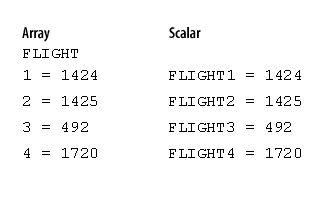Shell Variables   «Prev  Next»
 Lesson 10 Using array variables Objective Define how array variables are used in shell scripts.

# Using Array Variables in Shell Scripting

```agent1=555-99-1212
agent2=555-99-1213
agent3=555-99-1214
agent4=555-99-1215
```
It would be easy, however, if you could have one large variable that contained all the agents’ information, with an index to refer to a single piece of data. An array variable does this. You refer to an element of an array using an index number:
```agent=555-99-1212
agent=555-99-1213
agent=555-99-1214
agent=555-99-1215
```

This may look very similar to the first set of variables, but for large numbers of variables, arrays are much more efficient. Later in this course you will learn to use a loop with a counter. The counter can be used as the index number to access each element in a large array. Here is an example of how the array element is referred to:

```echo agent[\$COUNTER]
```

With regular variables, you must use the full name of each variable, so a loop cannot be used. The regular variables we have been using are calledscalar variables.
This diagram illustrates the conceptual difference between regular (scalar) variables and array variables.Regular (scalar) and array variables All of the elements of an array can be accessed at the same time by using the array index of @. For example, the following statement will print every element of the agent array.
```echo \${agent[@]}
```

### Using array variables

Unfortunately, the Bourne shell does not support array variables. You will not often need them in shell scripts, but if you do, the ksh, tcsh, and bash shells all support array variables.
In the next lesson, we will wrap up this module.

 Scalar: A standard or simple variable, as opposed to a variable that contains multiple values (like an array) or one that contains additional information (such as a vector in mathematical terms).